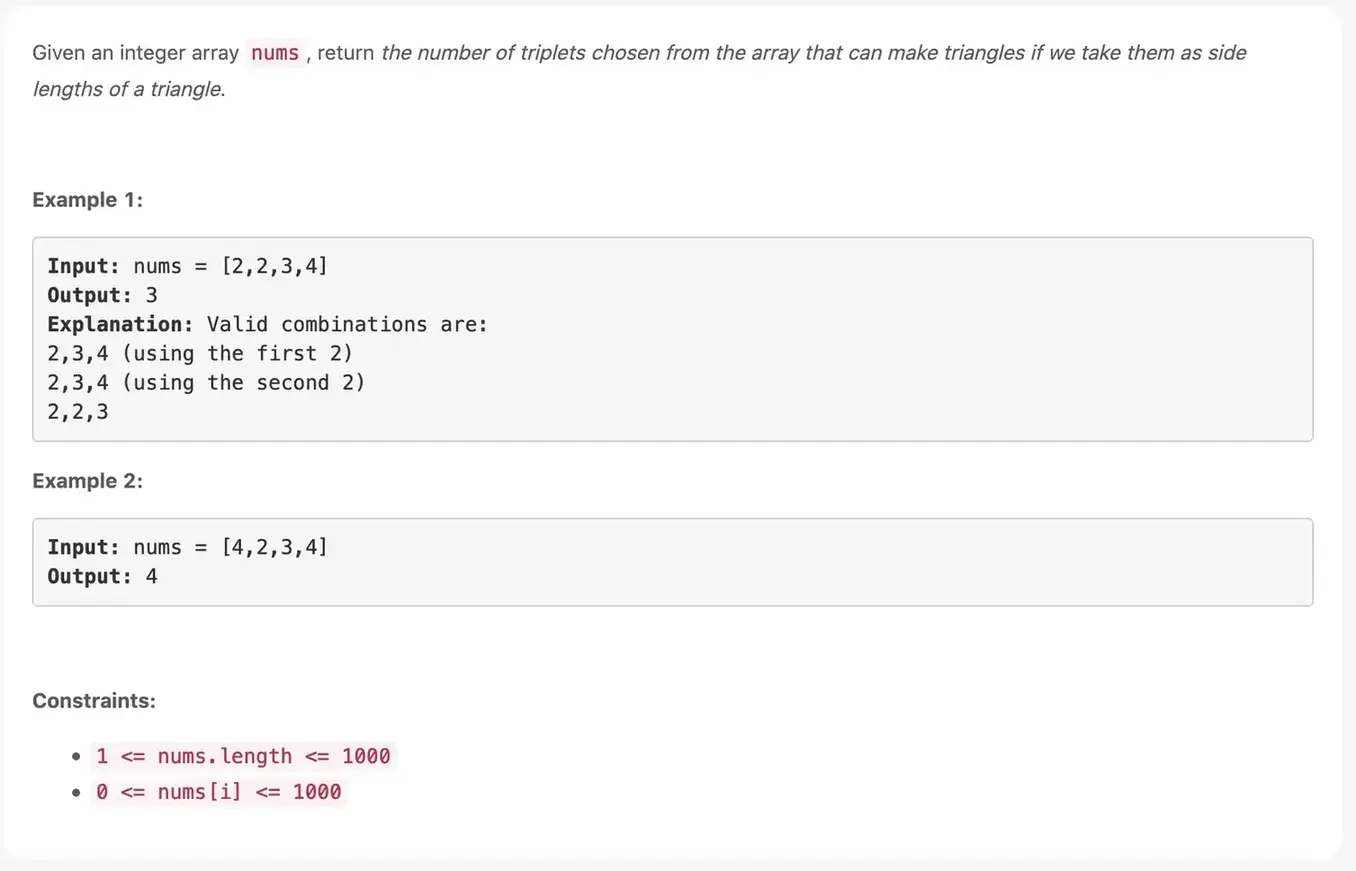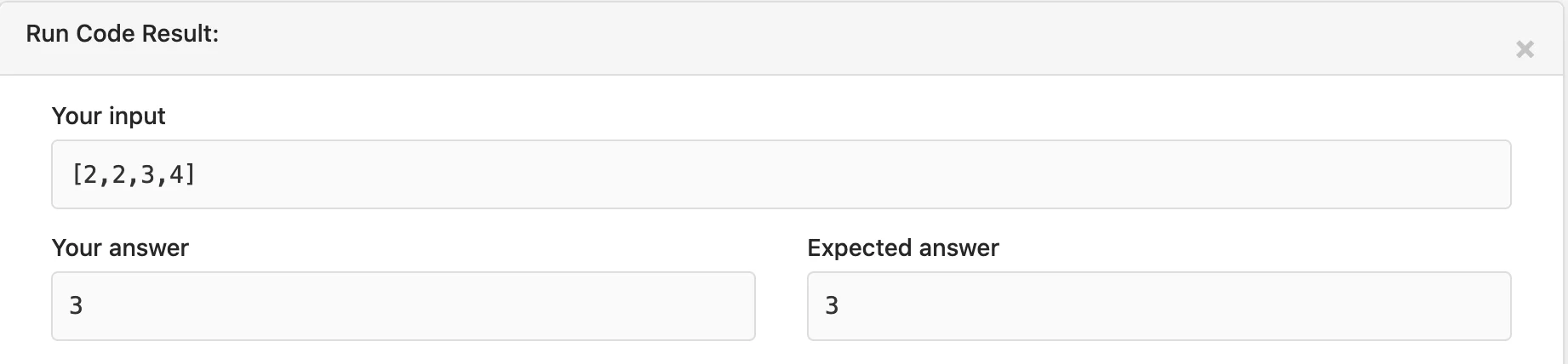# Valid Triangle Number

## Given an integer array nums, return the number of triplets chosen from the array that can make triangles if we take them as side lengths of a triangle.

Shubham Verma      July 27, 2021## Valid Triangle Number:

Given an integer array nums, return the number of triplets chosen from the array that can make triangles if we take them as side lengths of a triangle.

### Example 1:

Input: nums = [2,2,3,4]
Output: 3

### Explanation: Valid combinations are:

2,3,4 (using the first 2)
2,3,4 (using the second 2)
2,2,3

### Example 2:

Input: nums = [4,2,3,4]
Output: 4

### Constraints:

1 <= nums.length <=1000
0 <= nums[i] <=1000

### Solution:

``````                               /**
* @param {number[]} nums
* @return {number}
*/

var triangleNumber = function(arr) {
// Count of triangles
let count = 0;
let n = arr.length;

// The three loops select three
// different values from array
for (let i = 0; i < n; i++) {
for (let j = i + 1; j < n; j++) { // The innermost loop checks for // the triangle property
for (let k = j + 1; k < n; k++) // Sum of two sides is greater // than the third if ( arr[i] + arr[j]> arr[k]
&& arr[i] + arr[k] > arr[j]
&& arr[k] + arr[j] > arr[i])
count++;
}
}
return count;
};
``````

### Output:Valid Triangle Number

# For Other Countries (United States, United Kingdom, Spain, Italy, Germany, France, Canada, etc)

Thank you

To read more about the technologies, Please subscribe us, You'll get the monthly newsletter having all the published article of the last month.# 👈

## Enjoyed this moment?

### OTHER POSTS